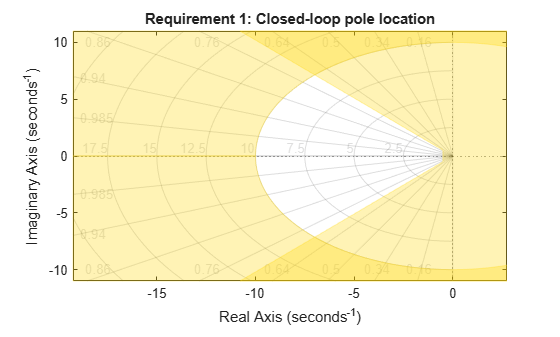# System Dynamics Specifications

This example shows how to constrain the poles of a control system tuned with `systune` or `looptune`.

The `systune` and `looptune` functions tune the parameters of fixed-structure control systems subject to a variety of time- and frequency-domain requirements. To specify these design requirements, use tuning goal objects.

### Closed-Loop Poles

The `TuningGoal.Poles` goal constrains the location of the closed-loop poles. You can enforce some minimum decay rate

`$Re\left(s\right)<-\alpha ,$`

impose some minimum damping ratio

`$Re\left(s\right)<-\zeta |s|,$`

or constrain the pole magnitude to

`$|s|<{\omega }_{max}.$`

For example

```MinDecay = 0.5; MinDamping = 0.7; MaxFrequency = 10; R = TuningGoal.Poles(MinDecay,MinDamping,MaxFrequency);```

constrains the closed-loop poles to lie in the white region below.

`viewGoal(R)`Increasing the `MinDecay` value results in faster transients. Increasing the `MinDamping` value results in better damped transients. Decreasing the `MaxFrequency` value prevents fast dynamics.

### Controller Poles

The `TuningGoal.ControllerPoles` goal constrains the pole locations for tuned elements such as filters and compensators. The tuning algorithm may produce unstable compensators for unstable plants. To prevent this, use the `TuningGoal.ControllerPoles` goal to keep the compensator poles in the left-half plane. For example, if your compensator is parameterized as a second-order transfer function,

`C = tunableTF('C',1,2);`

you can force it to have stable dynamics by adding the requirement

```MinDecay = 0; R = TuningGoal.ControllerPoles('C',MinDecay);```